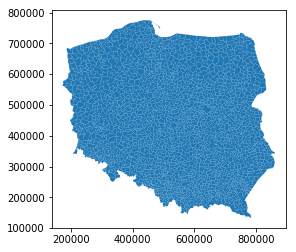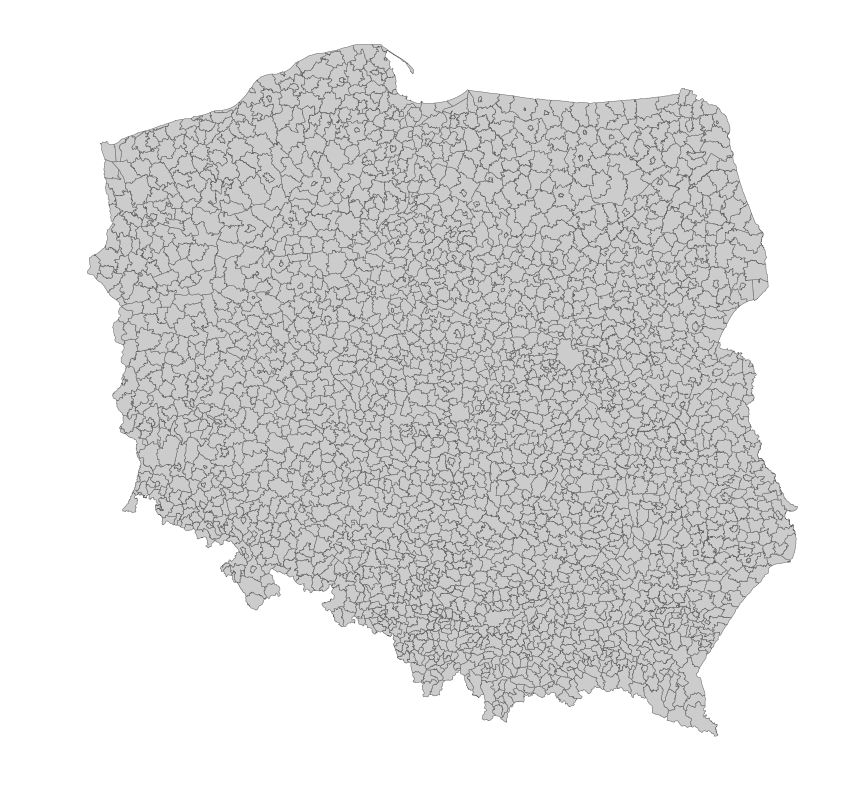# Maps and Python

Recently I've been working a lot with maps and geographical data. Most of the work has been dome in Stata and I've been using mostly QGIS for visualization. Also I did some simple spatial operations in GDAL and ArcGIS. But when it comes to producing maps, QGIS was my tool of choice. Ideas change quite a lot and QGIS is nice tool for data visualization, but unfortunately I've found it's Python API a bit troublesome to help me automatize production of maps. So I kept doing things manually.

## Tools¶

Here I turn to Python and its libraries to help me automatize map production. It turn out not much effort is needed and the maps being produced are visually appealing just after some minor tweaks.

In this short excercise I use Geopandas which enables a simple way to work with Geo data. Except that I also use the standard scientific libraries like Matplotlib, Pandas and Numpy.

## Data sources¶

In this example I use administrative boundaries for Poland and connect it with Local Data Bank, BDL data from the Polish national statistics office.

First we need to setup our environment. I always try to use absolute paths, so it's easy for me to switch between computers.

In :
%matplotlib inline
import matplotlib.pyplot as plt
import pandas as pd
import numpy as np
import geopandas as gpd

import os

# Project directory
pDir = "/home/mateusz/projects/gis/"


Openinig a Shapefiles is extremly easy. Geopandas documentation says that the only thing that you jus need one line of code to do that. Lets try reading the municipalities data...

In :
try:
pl_gminy = gpd.read_file(os.path.join(pDir, 'data', 'prg2018', 'gminy.shp'))
except ValueError as e:
print(e)

year 0 is out of range


Unfortunately I got an error. That's probably because the SHP files are created on Windows machines using Polish localization. The default encoding in use is the CP1250. To be able to read the municipalities file I had to open the original first in QGIS indicating the encoding and then save the file with UTF-8 encoding selected. It worked like a charm.

I also left just the columns with names and municipality codes (TERYT) jpt_kod_je.

In :
pl_gminy = gpd.read_file(os.path.join(pDir, 'data', 'prg2018', 'gminy_utf.shp'))

Out:
jpt_kod_je jpt_nazwa_ geometry
0 0222033 Wołów POLYGON ((319872.625979769 383951.756238694, 3...
1 0214062 Oleśnica POLYGON ((380436.400932976 368874.817494596, 3...
2 0223022 Długołęka POLYGON ((365569.581931299 376008.352757658, 3...
3 0214073 Syców POLYGON ((397867.372079491 383092.820273452, 3...
4 0223012 Czernica POLYGON ((370352.948437282 358781.965472659, 3...

So the geometry is coded in the geometry column. Nice to know. Let's see what is the projection in use.

In :
pl_gminy.crs

Out:
{'proj': 'tmerc',
'lat_0': 0,
'lon_0': 19,
'k': 0.9993,
'x_0': 500000,
'y_0': -5300000,
'ellps': 'GRS80',
'units': 'm',
'no_defs': True}

The used units are meters, so we can easily calculate area.

In :
pl_gminy['area'] = pl_gminy['geometry'].area
pl_gminy['area'].describe()

Out:
count    2.478000e+03
mean     1.261083e+08
std      7.858655e+07
min      3.312067e+06
25%      7.676658e+07
50%      1.117959e+08
75%      1.594393e+08
max      6.340781e+08
Name: area, dtype: float64
In :
np.sum(pl_gminy['area'])/1e6 # I divide by 1000x1000 m to get km^2

Out:
312496.4701659419

Google says that area of Poland is 312 679 km$^2$, so there is lack of precission here.

Let's try to plot the map.

In :
pl_gminy.plot()

Out:
<matplotlib.axes._subplots.AxesSubplot at 0x7f70891f5518>It worked, but the map still needs some minor improvements.

In :
fig, ax = plt.subplots(1, figsize=(14, 14))
pl_gminy.plot(ax=ax, linewidth=0.5, edgecolor='0.4', color='0.8')
ax.axis('off')

Out:
(137166.64559966073, 896406.6565782153, 101133.95416049592, 807108.9159837493)Much better! Lets try to add some color! First let's plot the area.

In :
fig, ax = plt.subplots(1, figsize=(14, 14))
pl_gminy.plot(ax=ax, edgecolor='0.4', linewidth=0.5,
column='area', cmap='Greens', legend=True, scheme='quantiles', k=5)
ax.axis('off')

Out:
(137166.64559966073, 896406.6565782153, 101133.95416049592, 807108.9159837493)